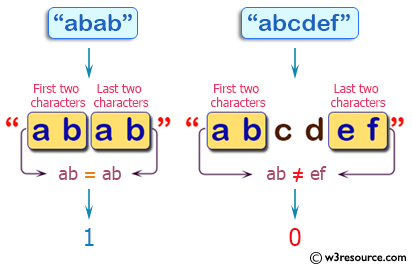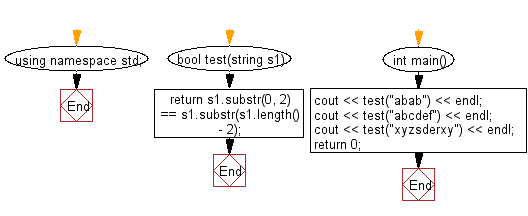﻿ C++ : If the first, last characters of a string are the same# C++ Exercises: Check whether the first two characters and last two characters of a given string are same

## C++ Basic Algorithm: Exercise-75 with Solution

Write a C++ program to check whether the first two characters and last two characters of a given string are same.

Sample Solution:

C++ Code :

``````#include <iostream>
using namespace std;

bool test(string s1)
{
return s1.substr(0, 2) == s1.substr(s1.length() - 2);
}

int main()
{
cout << test("abab") << endl;
cout << test("abcdef") << endl;
cout << test("xyzsderxy") << endl;
return 0;
}
``````

Sample Output:

```1
0
1
```

Pictorial Presentation:Flowchart:C++ Code Editor:

Contribute your code and comments through Disqus.

What is the difficulty level of this exercise?

﻿